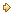Visitors Online: 77 | Tuesday 16th July 2019

CBSE Guess > Papers > Question Papers > Class XII > 2006 > Informatics Practices > Compartment Delhi Set - I

Informatics Practices — 2006 (Set I — Compartment Delhi)

SECTION - A

Q. 1. Answer the following questions:

1. Explain the term Business Computing with two examples. 2
2. What do you understand by the term UML? 2
3. What is Client Server Computing? 2
4. Give an example for one-to-one and one-to-many entity relation. 4

Q. 2. Answer the following questions:

1. Differentiate between Event Driven Programming and Object Oriented Programming. 2
2. What is Iteration? Name two statements which provide iteration in Visual Basic. 2
3. Explain the term ADO. 2
4. What is Variable Life Time? What is the Life Time of the following variables? 4
(i) Local variable
(ii) Public variable
(iii) Module variable

Q. 3. Answer the following questions:

1. Differentiate between Decision Control and Looping Control structure in context with PL/SQL. 4
2. What do you understand by the term Implicit Cursor in PL/SQL? 2
3. Differentiate between DBMS_OUTPUT.PUT_LINE ( ) and DBMS_ OUTPUT.PUT( ) statements. 2
4. Define a TRIGGER with two examples. 2

SECTION - B

Q. 4. Read the following case study and answer the questions given below:

ABC FINANCIAL company provides personal loans to customers on request. The programmer is assigned the responsibility of designing an EMI calculator. He decides to use Visual Basic to develop this and the following form is created.

The List for the above from is as follows:

 Object Type Object Name Description Form FrmEMICALC The Main Form Object Text Box xtName To enter name of the customer txtInterest To display the compound interest txtEMI To display Equal Monthly Instalment Option Buttons opt5years To select time as 5 years Opt10years To select time as 10 years Opt15years To select time as 15 years Command Buttons cmdCalculate To calculate interest and EMI cmdclear To clear all entered values cmdExit To end the application
1. Write the code to disable the text boxes txtlnterest and txtEMI. 2
2. Write cmdClear to clear all text boxes and set default choice in the option button as 5 years. Also set focus to txtLoanAmt. 2
3. Write code to assign the caption to Command Buttons cmdCalculate and cmdClear. 2
4. Write code for the Click event of the Command Button cmdCalculate to calculate the interest using the formula P * (1 + R) “T — P, where P is the loan amount, R is the interest rate (fixed as 11%), and T is the number of years. And the value of EMI is calculated as EM! = (Loan Amount + Interest) I (no. of years*12) 4

Q. 5. Answer the following questions:

1. The following code is to print all numbers which are squares but less than 50. Find the errors in the following code segment and rewrite the corrected code under lining the correction made. 2
Dim square As integer, num As Integer
num = 1
square num * num
Do
Print square
square = num * num
Loop square > = 50
2. Find the output of the following code segment: 2
For x = ASC (“A”) To ASC (“E”)
For y ASC (“A”) to x
Print Chr(y);
Next y
Print
Next x
3. Rewrite the following code using If ... Else instead of Select Case. 2
Select Case          Round (Avg)
Case Is = 100
Case 75 To 99
Case 60 To 74
Case 50 To 59
Case Is <50
End Select
4. Write a Visual Basic procedure which will take two integer values X and Y as arguments and display the following : 4
(i) X to the power of Y
(ii) The highest number among X and Y

SECTION - C

1. Write the output produced by the following part of code in PL/SQL: 2
DECLARE
Num NUMBER;
V NUMBER : = 0;
BEGIN
For Num IN 2 ...5
LOOP
V : = V + Num * Num;
DBMS_OUTPUT.PUT_LINE(V);
END LOOP;
END;
2. Refer the following table STUDENT and give the output produced by the PL/SQL code given: 2
TABLE : STUDENT
 NAME AGE MARKS RAJA SIMON WILSON MARY 17 16 18 17 325 480 390 450
DECLARE
T_NAME VARCHAR2(1 0);
T_AGE NUMBER(2);
T_MARKS NUMBER(3);
BEGIN
T_AGE : = 17;
SELECT MARKS INTO T MARKS FROM STUDENT
WHERE AGE > T_AGE;
IF T_MARKS > 350
DBMS_OUTPUT.PUT_LINE (T_MARKS);
END;
3. Differentiate between Scalar and composite data type in PL/SQL. 2
4. Write a PL/SQL function chkPrime that takes one number as argument and returns a value 1 if the number passed is Prime else will return a value zero. 4

Q. 7. Answer the questions based on the table CARS given below:

TABLE : CARS

 Column Name Data Type Size Constraint Description Model_No VARCHAR2 20 Primary Key Model number of the car Manufacturer VARCHAR2 20 NOT NULL Name of the manufacturer Colour VARCHAR2 10 Colour of the car Price NUMBER 8 Price of the car Capacity NUMBER 5 Engine capacity
1. Write SQL command to create the table CARS including the constraints. 2
2. Write the SQL command to display the details of all cars whose colour is the same as the colour of Model_No M_800. 2
3. Write PL/SQL code to increase the Price of all cars by 5%. 2
4. ) Write FL/SQL block to delete all cars with a particular Model Number from the table CARS, where the value of Model Number is accepted from the user. 4

 Informatics Practicess 2006 Question Papers Class XII Delhi Outside Delhi Compartment DelhiSet ISet ISet I

CBSE 2006 Question Papers Class XII